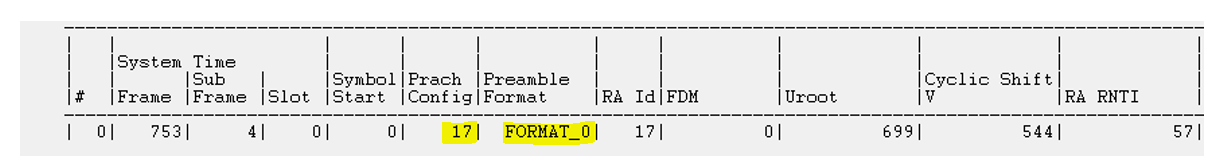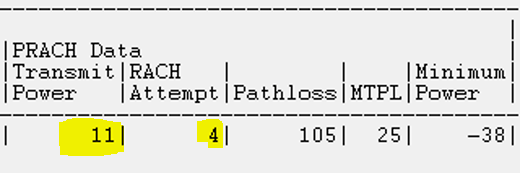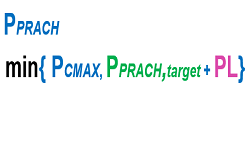# 5G NR PRACH Power Control: MSG1 Tx Power – Open Loop Power

In any wireless System, when a device (UE) needs to get access  it has to send a signal or preamble (MSG1) to base station (gNB), then energy is needed. One might have question, at what power it needs to send the preamble so that it is detected successfully ?  The 3GPP specification, 38.213 gives the  following formula about PRACH power control calculation.The formula says RACH power shall be minimum of two, Pcmax (i) or PRACH Target + PL (ii). Pcmax is depend on UE category and usually consider as 23dBm. PPRACH power use Open Loop power control, which mean base station only indicate UE for target power and does not control further with TPC commands.

Step 1:  Calculate PPRACH, target power  using following formula.• DELTA_PREAMBLE can be obtained using prach-configuration Index translation to preamble format and then lookup in following table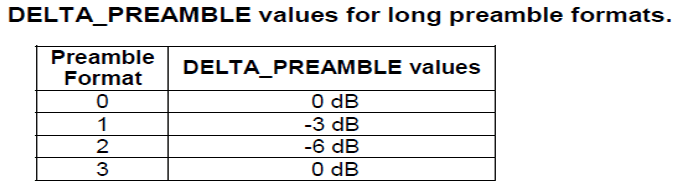• PREAMBLE_POWER_RAMPING_COUNTER is 1 for the initial transmission, and the retransmission is incremented by one each time
• PREAMBLE_POWER_RAMPING_STEP is derived from the RRC parameter “powerRampingStep”

Step#2 Then, calculate Path Loss (PL) using following formula• referenceSignalPower is determined by the parameters ss-PBCH-BlockPower and powerControlOffsetSS according to the SSB or CSI-RS associated with PRACH
• HigherlayerfilteredRSRP is the RSRP measured when sending PRACH

Example #1:

We have following RACH parameter received as part of RRC reconfiguration message within rach-ConfigGeneric.Assuming following RSRP at the time of RACH procedure is -85dBm

Step: 2 Calculating path loss

• PL= refence signal power – RSRP (from above log ss-PBCH-BlockPower= -12)
• PL= -12 – (-85) = 73 dB

Step 1: PRACH Target Power

• prach-Configuration Index is associated with preamble format —-> 0 so DELTA_PREAMBLE —-> 0 dBm
• preambleReceivedTargetPower as per logs is -100dBm
• powerRampingStep as per logs is 4dB
• PRACH target = -100 + 0 +(1-1)*4 = -100 dBm

Step 0: Calculating PRACH Tx Power

• PRACH Tx Power = min {Pcmax , PRACH Target + PL } dBm
• PRACH Tx Power = min {23 , -100 + 73 } dBm
• PRACH Tx Power = min {23 , -27 } dBm
• -27 dBm

So UE will transmit the RACH with -27 dBm power at first time. If RACH procedure fails at Msg2 or Msg4 fails, UE will trigger Msg1 retransmission. The maximum number of Msg1 retransmissions is defined by the parameter preambleTransMax . If a retransmission of Msg1 occurs, the power of each retransmission will increase by the step of powerRampingStep which is 4dBm as per above log snippet until UE reach the maximum power or the maximum number of retransmissions is reached. It should be noted here that the retransmission to increase the power is conditional, given that the SSB associated with  PRACH has not changed.

Example#2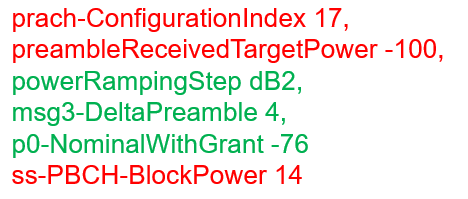• prach-ConfigurationIndex 17 —-> preamble format 0 —–>  DELTA_PREAMBLE = 0dB